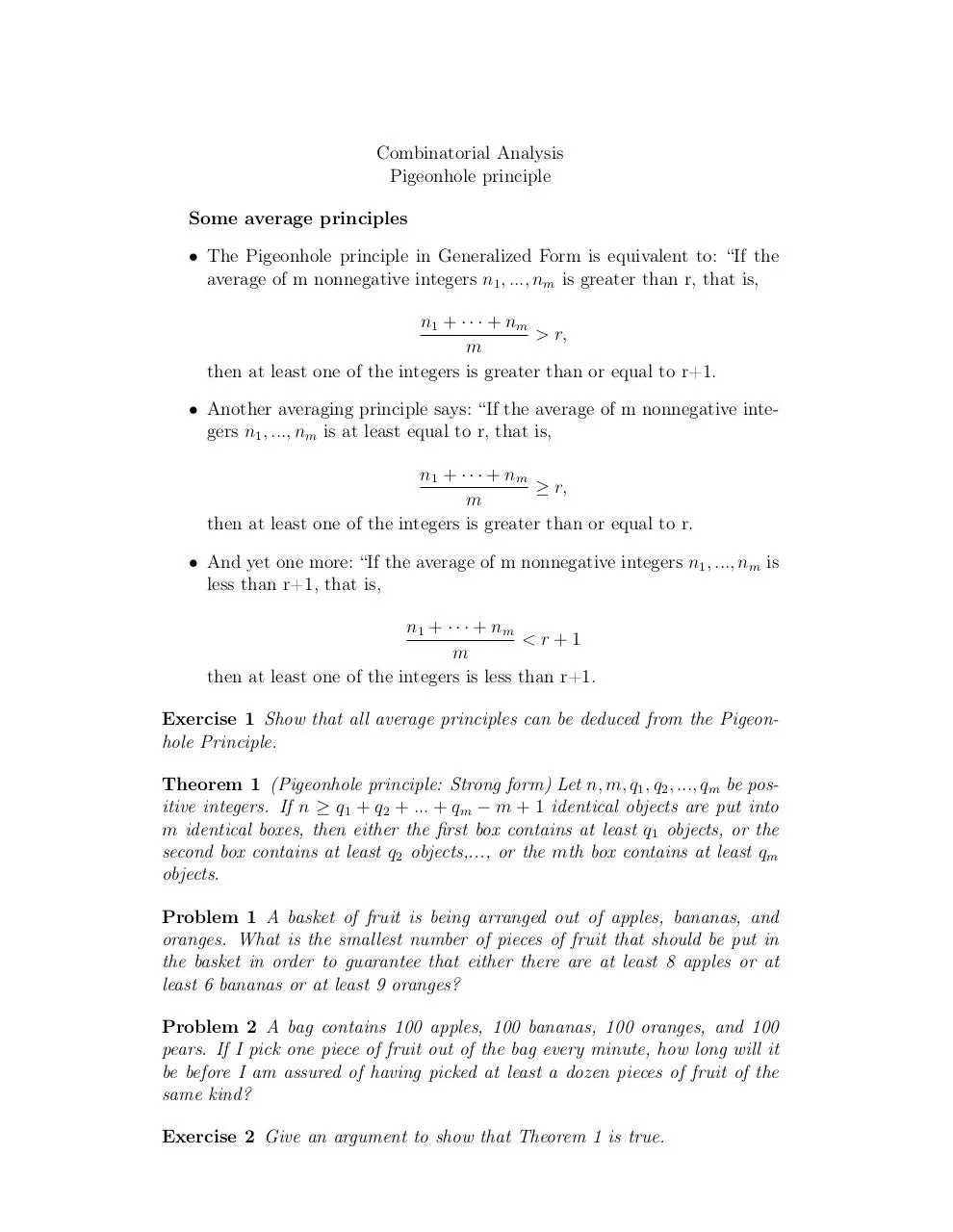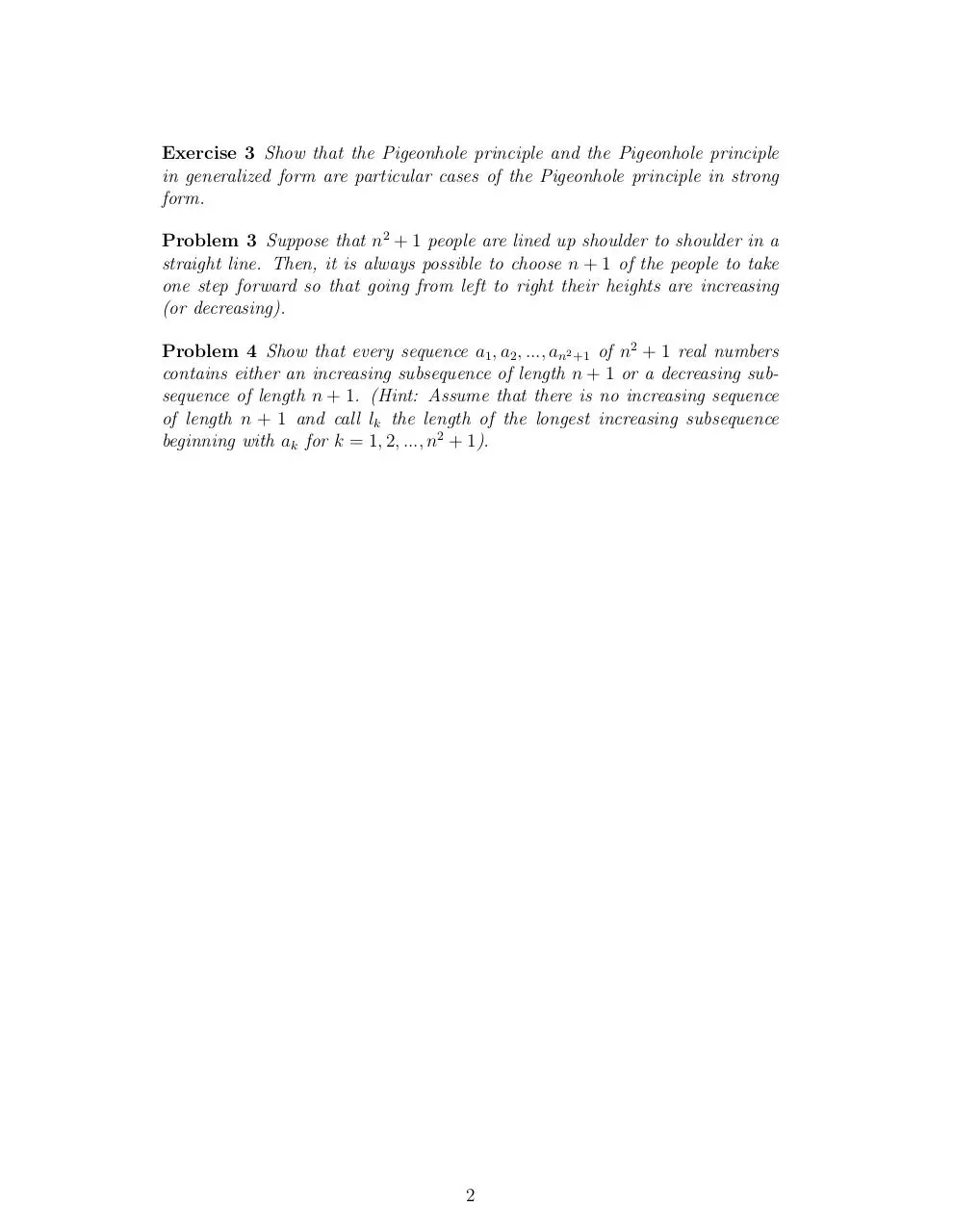# Day 4 Pigeonhole .pdf

### File information

This PDF 1.4 document has been generated by TeX / pdfTeX-1.40.3, and has been sent on pdf-archive.com on 24/01/2014 at 06:06, from IP address 169.231.x.x. The current document download page has been viewed 1139 times.
File size: 89.45 KB (2 pages).
Privacy: public file

### Document preview

Combinatorial Analysis
Pigeonhole principle
Some average principles
• The Pigeonhole principle in Generalized Form is equivalent to: “If the
average of m nonnegative integers n1 , ..., nm is greater than r, that is,
n1 + · · · + nm
&gt; r,
m
then at least one of the integers is greater than or equal to r+1.
• Another averaging principle says: “If the average of m nonnegative integers n1 , ..., nm is at least equal to r, that is,
n1 + · · · + nm
≥ r,
m
then at least one of the integers is greater than or equal to r.
• And yet one more: “If the average of m nonnegative integers n1 , ..., nm is
less than r+1, that is,
n1 + · · · + nm
&lt;r+1
m
then at least one of the integers is less than r+1.
Exercise 1 Show that all average principles can be deduced from the Pigeonhole Principle.
Theorem 1 (Pigeonhole principle: Strong form) Let n, m, q1 , q2 , ..., qm be positive integers. If n ≥ q1 + q2 + ... + qm − m + 1 identical objects are put into
m identical boxes, then either the first box contains at least q1 objects, or the
second box contains at least q2 objects,..., or the mth box contains at least qm
objects.
Problem 1 A basket of fruit is being arranged out of apples, bananas, and
oranges. What is the smallest number of pieces of fruit that should be put in
the basket in order to guarantee that either there are at least 8 apples or at
least 6 bananas or at least 9 oranges?
Problem 2 A bag contains 100 apples, 100 bananas, 100 oranges, and 100
pears. If I pick one piece of fruit out of the bag every minute, how long will it
be before I am assured of having picked at least a dozen pieces of fruit of the
same kind?
Exercise 2 Give an argument to show that Theorem 1 is true.

Exercise 3 Show that the Pigeonhole principle and the Pigeonhole principle
in generalized form are particular cases of the Pigeonhole principle in strong
form.
Problem 3 Suppose that n2 + 1 people are lined up shoulder to shoulder in a
straight line. Then, it is always possible to choose n + 1 of the people to take
one step forward so that going from left to right their heights are increasing
(or decreasing).
Problem 4 Show that every sequence a1 , a2 , ..., an2 +1 of n2 + 1 real numbers
contains either an increasing subsequence of length n + 1 or a decreasing subsequence of length n + 1. (Hint: Assume that there is no increasing sequence
of length n + 1 and call lk the length of the longest increasing subsequence
beginning with ak for k = 1, 2, ..., n2 + 1).

2Day 4-Pigeonhole.pdf (PDF, 89.45 KB)

### Share on social networks

#### HTML Code

Copy the following HTML code to share your document on a Website or Blog